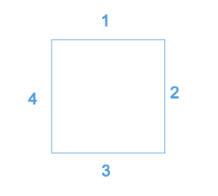SSAT Elementary Level Math : How to find the perimeter of a square

Example Questions

Example Question #11 : How To Find The Perimeter Of A Square

A square has an area of 49 square units. What is its perimeter?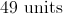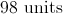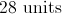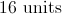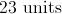Explanation:

The area of a square is the side length squared.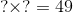We can find this number by taking the square root of 49.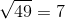Therefore, the length of each side is 7 units.

The perimeter of a square is the sum of the lengths of the sides. There are 4 sides, each with a length of 7.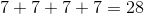The perimeter is 28 units.

Example Question #12 : How To Find The Perimeter Of A Square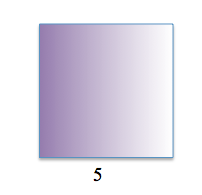What is the perimeter of the above square?Explanation:

To find the perimeter of the square, add all four sides together. Even though we are not given the length of all four sides, we know each side is the same length because all squares have four equal sides.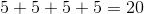Example Question #13 : How To Find The Perimeter Of A Square

If the area of a square is, what is the perimeter of the square?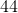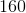Explanation:

Write the formula for the area of a square and substitute the area.  The side length is needed to find the perimeter.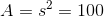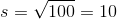There aresides in a square.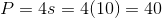Example Question #14 : How To Find The Perimeter Of A Square

The perimeter of a square is. What is the length of one of its sides?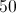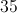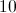Explanation:

A square has four equal sides. Perimeter is the sum of these four sides. Therefore,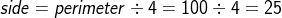.

Example Question #15 : How To Find The Perimeter Of A Square

What is the PERIMETER of the square in the figure?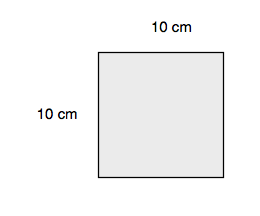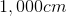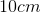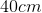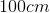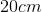Explanation:

Because the problem asks you to find the perimeter, you are looking for the total distance around the outside of the shape. The problem also tells you that the figure is a square, so you know that all the sides are 10 cm. Adding all four sides gives the perimeter: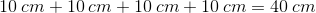Example Question #16 : How To Find The Perimeter Of A Square

If the perimeter of a square is, what is the length of each side?Explanation:

Since all four sides of a square have the same length, we can multiply the length byto find the perimeter: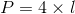Plug in the perimeter and solve for the unkown length: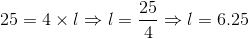Example Question #17 : How To Find The Perimeter Of A Square

What is the perimeter of a square with an area of?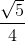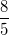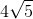Explanation:

Write the area of the square.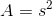Substitute the area and solve for the square's side.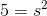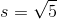Since there are four equal sides in a square, multiply the side by four.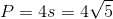Example Question #18 : How To Find The Perimeter Of A Square

Find the perimeter whose side length is 5.Explanation:

To solve, simply use the formula for area.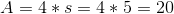Example Question #19 : How To Find The Perimeter Of A Square

Find the perimeter of a square whose side length is 11.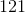Explanation:

To find perimeter of a square, simply use the formula below: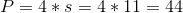Example Question #20 : How To Find The Perimeter Of A Square

Which shape has onlysides?

Cube

Triangle

Square

Hexagon

Pentagon

A square hassides.